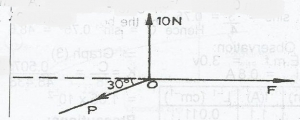The diagram above illustrates three forces acting on an object at point O. If the object is in equilibrium, determine the magnitude of the force P.

A. 10.5N
B. 11.0N
C. 17.3N
D. 20.0N